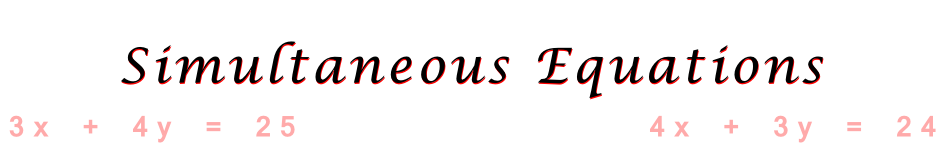There are 366 different Starters of The Day, many to choose from. You will find below some starters on the topic of Simultaneous Equations. A lesson starter does not have to be on the same topic as the main part of the lesson or the topic of the previous lesson. It is often very useful to revise or explore other concepts by using a starter based on a totally different area of Mathematics.

Main Page

Simultaneous Equations Starters:Work out the contents and the cost of the Christmas boxes from the given cluesA lamp and a bulb together cost 32 pounds. The lamp costs 30 pounds more than the bulb. How much does the bulb cost?Find the dimensions of a rectangle given the perimeter and area.Five numbers are added together in pairs and the sums shown. What might the five numbers be?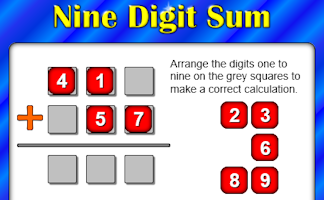The sum and product are given, can you find the two numbers?There are some rabbits and chickens in a field. Calculate how many of each given the number of heads and feet.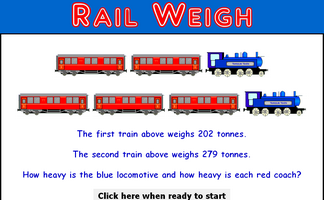Record the weights of the trains to work out the weight of a locomotive and a coach. A real situation which produces simultaneous equations.It is called Refreshing Revision because every time you refresh the page you get different revision questions.A problem involving two people's ages which can be solved using algebra.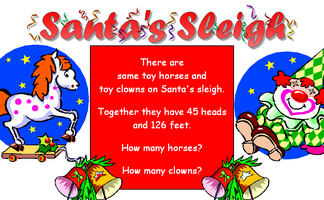Work out the number of clowns and horses given the number of heads and feet.A problem which can best be solved as a pair of simultaneous equations.Each traffic sign stands for a number. Some of the sums of rows and columns are shown. What numbers might the signs stand for?How many children and how many donkeys are on the beach? You can work it out from the number of heads and the number of feet!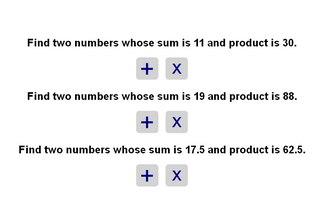Find the two numbers whose sum and product are given.

Advanced Simultaneous Equations Starters

Search

The activity you are looking for may have been classified in a different way from the way you were expecting. You can search the whole of Transum Maths by using the box below.

Have today's Starter of the Day as your default homepage. Copy the URL below then select
Tools > Internet Options (Internet Explorer) then paste the URL into the homepage field.

Set as your homepage (if you are using Internet Explorer)

Do you have any comments? It is always useful to receive feedback and helps make this free resource even more useful for those learning Mathematics anywhere in the world. Click here to enter your comments.For All: